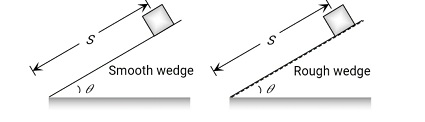# Then find the Coefficient of Friction between body and wedge in term of n?

For the below figureThe distance S is the same.

And using this concept we get

Where

coefficient of friction

Angle of inclination

n = an integer

Exams
Articles
Questions